安卓 OpenGL ES 2.0 完全入门（三）：2D 纹理的裁剪、翻转、旋转、缩放

Posted by Piasy on October 6, 2017

整体思路

裁剪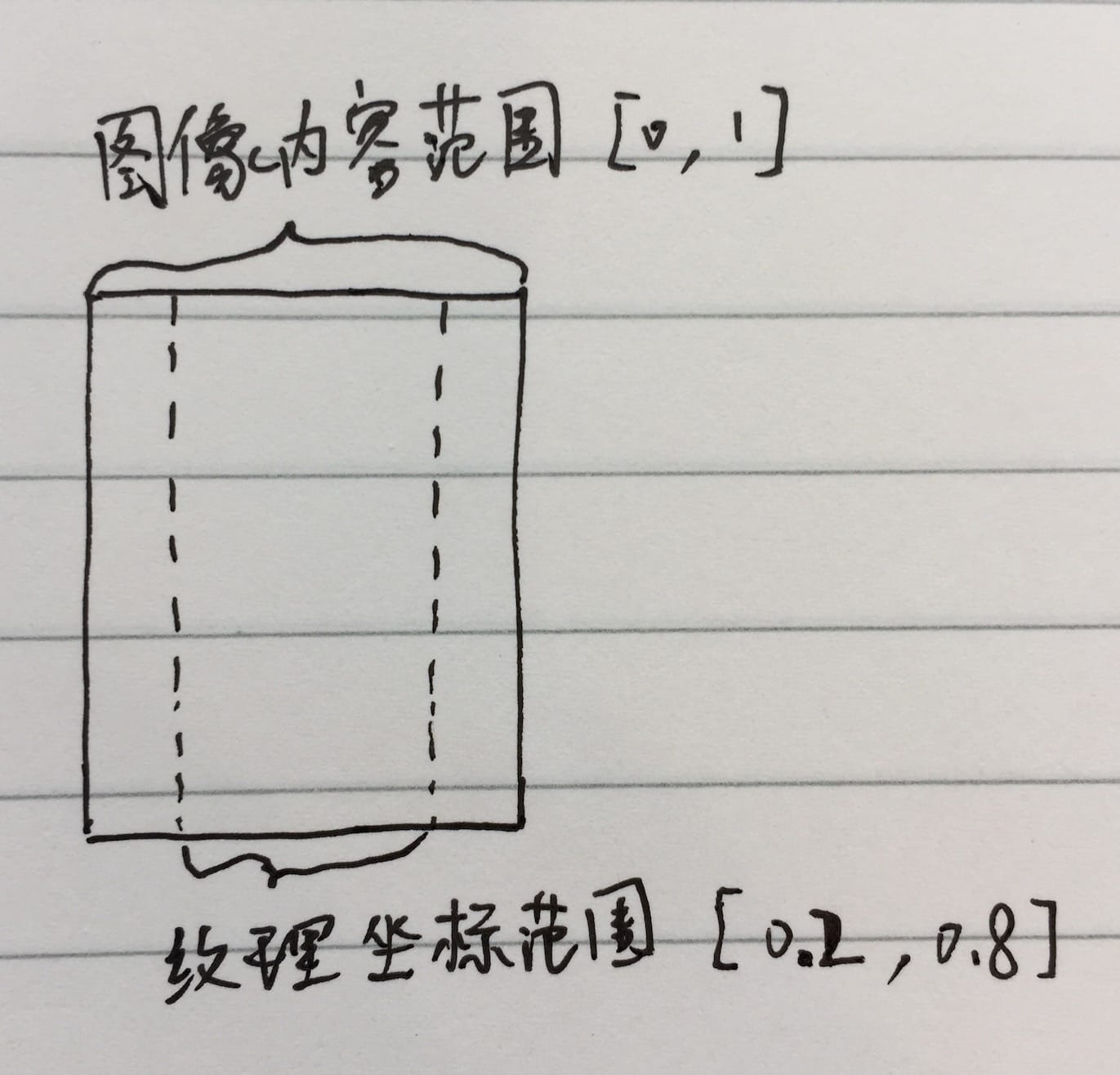旋转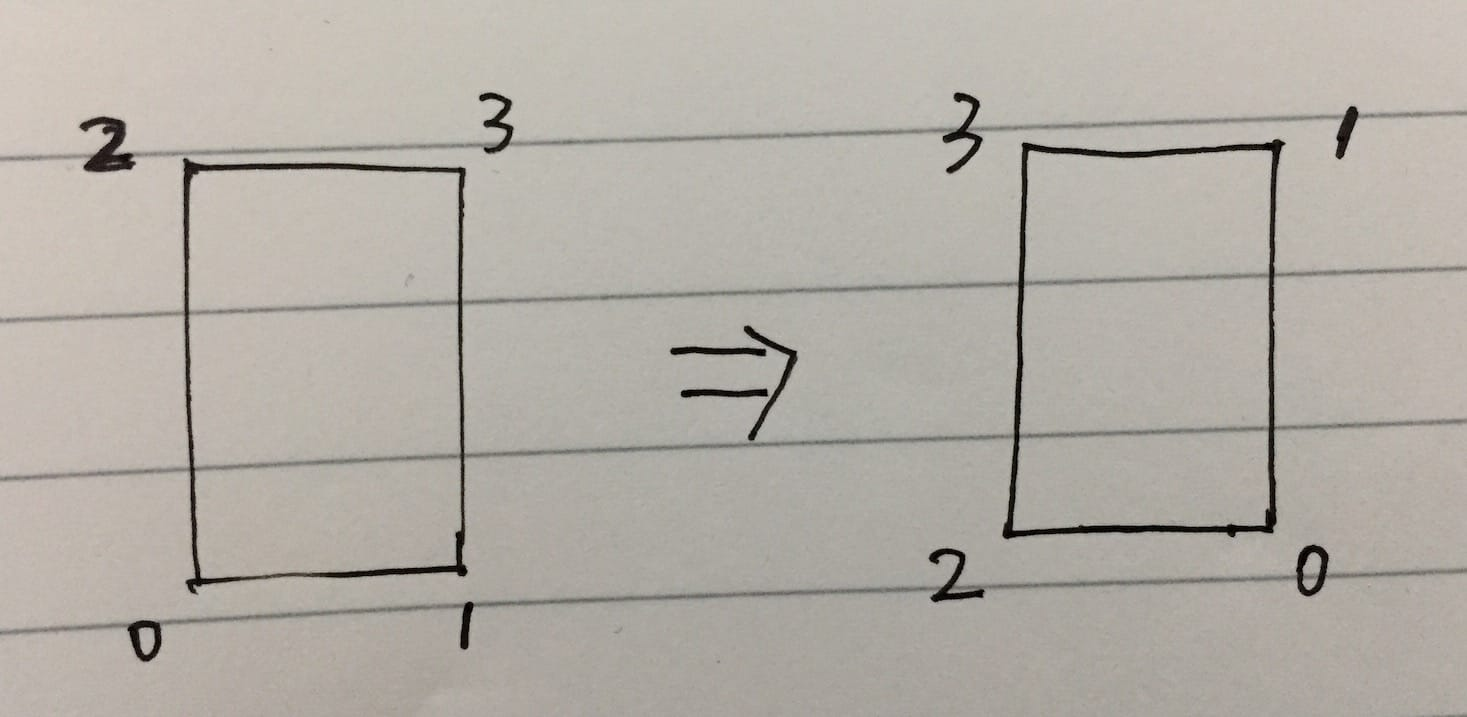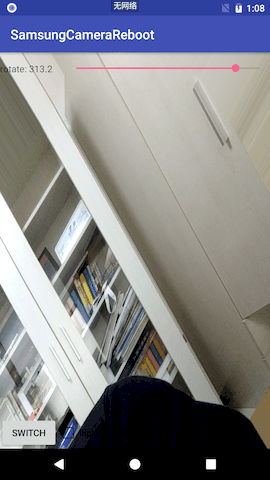缩放

• FIT_XY：宽高都填充满，如果宽高比不一致，则会发生变形；
• CENTER_CROP：短边填充满，长边等比例缩放，超出部分两端裁掉；
• CENTER_INSIDE：长边填充满，短边等比例缩放，不足部分两端留黑边；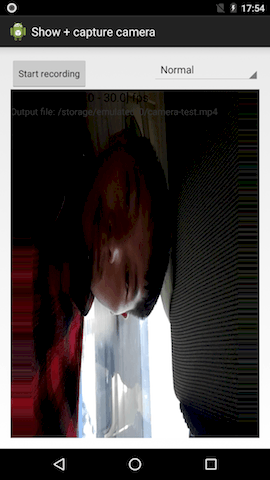关于条纹状效果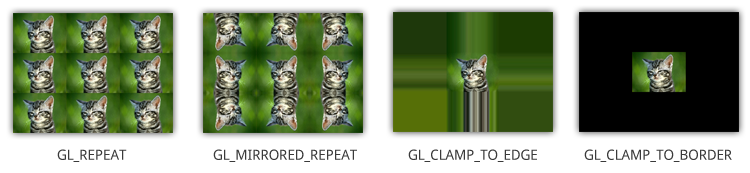Show me the code

Talk is cheap, show me the code.

Grafika 的绘制逻辑封装在 Texture2dProgram#draw 函数中，其 Shader 代码非常简单，已经基本被矩形、图片、读取显存等涵盖了，只是绘制矩形时用了另一种接口。我们之前使用的是 GLES20.glDrawElements(GLES20.GL_TRIANGLES,,,)，而 Grafika 使用的是 GLES20.glDrawArrays(GLES20.GL_TRIANGLE_STRIP,,)

glDrawElements 是给定顶点列表和绘制顺序（顶点索引列表）进行绘制，而 glDrawArrays 则只给定顶点列表。对于非常复杂的模型，需要很多三角形构成，顶点大量重复，而顶点坐标占用的空间比索引占用的空间大得多，因此 glDrawElements 空间效率会更高。

• GL_TRIANGLES：每三个顶点构成一个三角形，即顶点 012 构成一个三角形，顶点 345 构成一个三角形，依此类推；
• GL_TRIANGLE_STRIP：任意相邻三个顶点都构成一个三角形，即顶点 012 构成一个三角形，顶点 123 构成一个三角形，依此类推；
• GL_TRIANGLE_FAN：首个顶点不动，后续任意相邻两个顶点与首个顶点构成一个三角形，即顶点 012 构成一个三角形，顶点 023 构成一个三角形，依此类推；

处理框架

private static final float FULL_RECTANGLE_COORDS[] = {
-1.0f, -1.0f,   // 0 bottom left
1.0f, -1.0f,    // 1 bottom right
-1.0f,  1.0f,   // 2 top left
1.0f,  1.0f,    // 3 top right
};
private static final float FULL_RECTANGLE_TEX_COORDS[] = {
0.0f, 0.0f,     // 0 bottom left
1.0f, 0.0f,     // 1 bottom right
0.0f, 1.0f,     // 2 top left
1.0f, 1.0f      // 3 top right
};

public void setTransformation(final Transformation transformation) {
if (mPrefab != Prefab.FULL_RECTANGLE) {
return;
}

vertices = Arrays.copyOf(FULL_RECTANGLE_COORDS, FULL_RECTANGLE_COORDS.length);
textureCoords = new float;

if (transformation.cropRect != null) {
resolveCrop(transformation.cropRect.x, transformation.cropRect.y,
transformation.cropRect.width, transformation.cropRect.height);
} else {
resolveCrop(Transformation.FULL_RECT.x, Transformation.FULL_RECT.y,
Transformation.FULL_RECT.width, Transformation.FULL_RECT.height);
}
resolveFlip(transformation.flip);
resolveRotate(transformation.rotation);
if (transformation.inputSize != null && transformation.outputSize != null) {
resolveScale(transformation.inputSize.width, transformation.inputSize.height,
transformation.outputSize.width, transformation.outputSize.height,
transformation.scaleType);
}

mVertexArray = GlUtil.createFloatBuffer(vertices);
mTexCoordArray = GlUtil.createFloatBuffer(textureCoords);
}

resolveCrop

private void resolveCrop(float x, float y, float width, float height) {
float minX = x;
float minY = y;
float maxX = minX + width;
float maxY = minY + height;

// left bottom
textureCoords = minX;
textureCoords = minY;
// right bottom
textureCoords = maxX;
textureCoords = minY;
// left top
textureCoords = minX;
textureCoords = maxY;
// right top
textureCoords = maxX;
textureCoords = maxY;
}

resolveFlip

private void resolveFlip(int flip) {
switch (flip) {
case Transformation.FLIP_HORIZONTAL:
swap(textureCoords, 0, 2);
swap(textureCoords, 4, 6);
break;
case Transformation.FLIP_VERTICAL:
swap(textureCoords, 1, 5);
swap(textureCoords, 3, 7);
break;
case Transformation.FLIP_HORIZONTAL_VERTICAL:
swap(textureCoords, 0, 2);
swap(textureCoords, 4, 6);

swap(textureCoords, 1, 5);
swap(textureCoords, 3, 7);
break;
case Transformation.FLIP_NONE:
default:
break;
}
}

resolveRotate

private void resolveRotate(int rotation) {
float x, y;
switch (rotation) {
case Transformation.ROTATION_90:
x = textureCoords;
y = textureCoords;
textureCoords = textureCoords;
textureCoords = textureCoords;
textureCoords = textureCoords;
textureCoords = textureCoords;
textureCoords = textureCoords;
textureCoords = textureCoords;
textureCoords = x;
textureCoords = y;
break;
case Transformation.ROTATION_180:
swap(textureCoords, 0, 6);
swap(textureCoords, 1, 7);
swap(textureCoords, 2, 4);
swap(textureCoords, 3, 5);
break;
case Transformation.ROTATION_270:
x = textureCoords;
y = textureCoords;
textureCoords = textureCoords;
textureCoords = textureCoords;
textureCoords = textureCoords;
textureCoords = textureCoords;
textureCoords = textureCoords;
textureCoords = textureCoords;
textureCoords = x;
textureCoords = y;
break;
case Transformation.ROTATION_0:
default:
break;
}
}

resolveScale

private void resolveScale(int inputWidth, int inputHeight, int outputWidth,
int outputHeight, int scaleType) {
if (scaleType == Transformation.SCALE_TYPE_FIT_XY) {
// The default is FIT_XY
return;
}

// Note: scale type need to be implemented by adjusting
// the vertices (not textureCoords).
if (inputWidth * outputHeight == inputHeight * outputWidth) {
// Optional optimization: If input w/h aspect is the same as output's,
// there is no need to adjust vertices at all.
return;
}

float inputAspect = inputWidth / (float) inputHeight;
float outputAspect = outputWidth / (float) outputHeight;

if (scaleType == Transformation.SCALE_TYPE_CENTER_CROP) {
if (inputAspect < outputAspect) {
float heightRatio = outputAspect / inputAspect;
vertices *= heightRatio;
vertices *= heightRatio;
vertices *= heightRatio;
vertices *= heightRatio;
} else {
float widthRatio = inputAspect / outputAspect;
vertices *= widthRatio;
vertices *= widthRatio;
vertices *= widthRatio;
vertices *= widthRatio;
}
} else if (scaleType == Transformation.SCALE_TYPE_CENTER_INSIDE) {
if (inputAspect < outputAspect) {
float widthRatio = inputAspect / outputAspect;
vertices *= widthRatio;
vertices *= widthRatio;
vertices *= widthRatio;
vertices *= widthRatio;
} else {
float heightRatio = outputAspect / inputAspect;
vertices *= heightRatio;
vertices *= heightRatio;
vertices *= heightRatio;
vertices *= heightRatio;
}
}
}

CENTER_CROP 时，如果 inputAspect < outputAspect，即输入宽高比小于输出宽高比，则说明（竖屏时）输入宽是短边高是长边，宽填充满即顶点横坐标取值范围充满 [-1, 1]，高按比例放大即各顶点纵坐标值乘以放大系数，系数为 outputAspect / inputAspect（推理过程见下图）。inputAspect > outputAspect 说明高是长边宽是短边，其处理逻辑同理可得。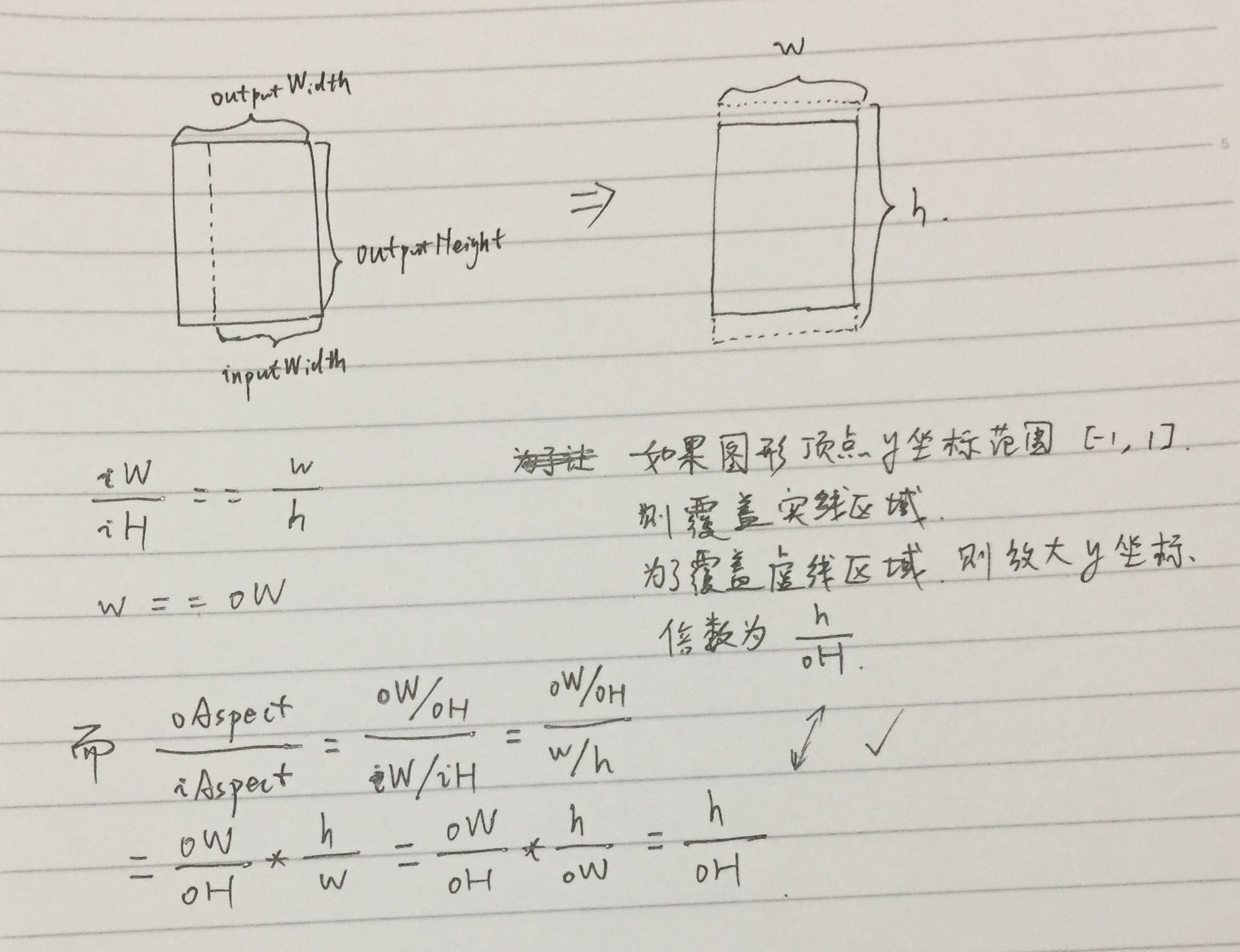CENTER_INSIDE 时，ratio 的计算结果小于 1，即限制顶点坐标的取值范围，使其小于 [-1, 1]，其逻辑类似，大家可以自行推导。

总结